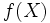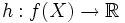# Pseudocompactness is continuous image-closed

This article gives the statement, and possibly proof, of a topological space property (i.e., pseudocompact space) satisfying a topological space metaproperty (i.e., continuous image-closed property of topological spaces)
View all topological space metaproperty satisfactions | View all topological space metaproperty dissatisfactions
Get more facts about pseudocompact space |Get facts that use property satisfaction of pseudocompact space | Get facts that use property satisfaction of pseudocompact space|Get more facts about continuous image-closed property of topological spaces

## Statement

Suppose$X$ is a topological space and$f:X \to Y$ is a continuous map. Then, if$X$ is a pseudocompact space, the image$f(X)$ (endowed with the subspace topology from$Y$) is also a pseudocompact space.

## Proof

Given: A topological space$X$ such that, for any continuous map$g:X \to \R$,$g(X)$ is bounded. A continuous map$f:X \to Y$.

To prove: For any continuous map$h:f(X) \to \R$,$h(f(X))$ is bounded.

Proof: Note first that since$f:X \to Y$ is continuous, so is$f:X \to f(X)$. Henceforth, we think of$f$ as a map from$X$ to$f(X)$.

Consider a continuous map$h:f(X) \to \R$. Define$g = h \circ f$. Then,$g:X \to \R$ is a map. Since both$h$ and$f$ are continuous, so is$g$, and by assumption, we therefore have$g(X)$ bounded. But$g(X) = h(f(X))$ by definition, so$h(f(X))$ is bounded, completing the proof.